Home > CCA2 > Chapter 8 > Lesson 8.2.2 > Problem8-94

8-94.
1.Match each equation with the appropriate graph. Do this without using a graphing calculator. Homework Help ✎

1. y = sin(x +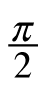)

2. y = sin(2x)

3. y = 2sin(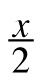)

4. y = sin(x) − 3

5. y = −sin[2(x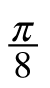)]

2.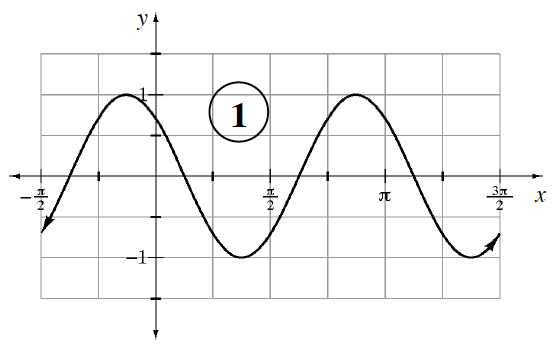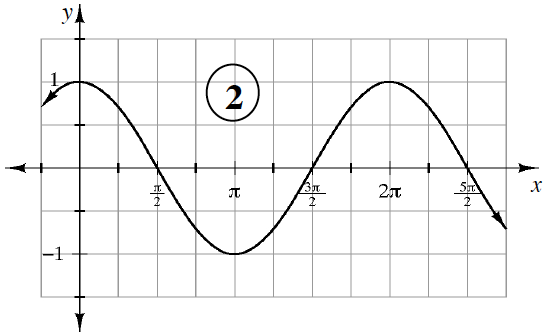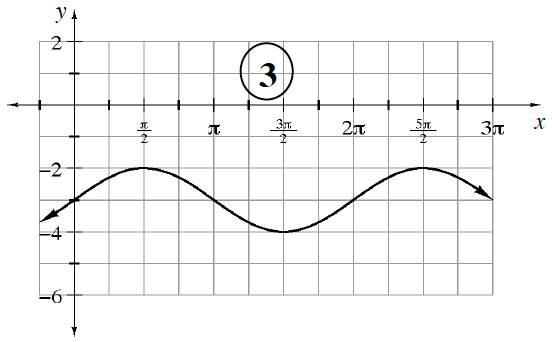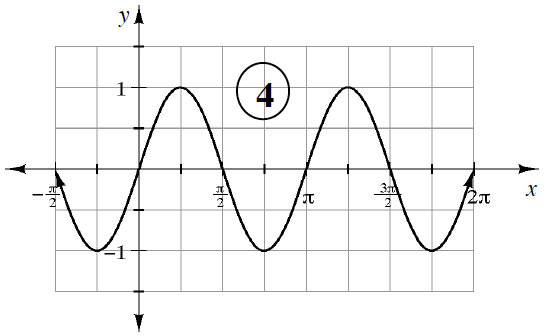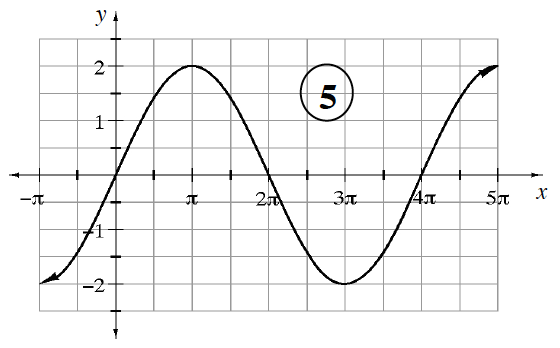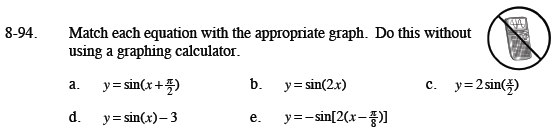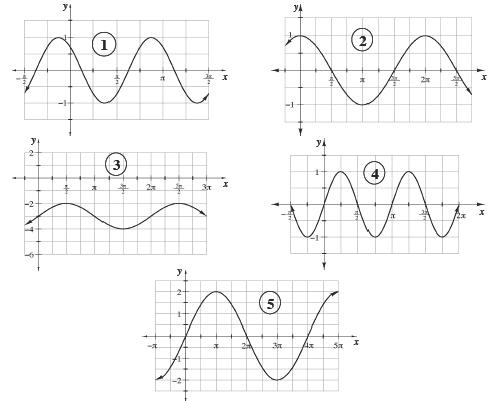(a) 2
(b) 4
(c) 5
(d) 3
(e) 1